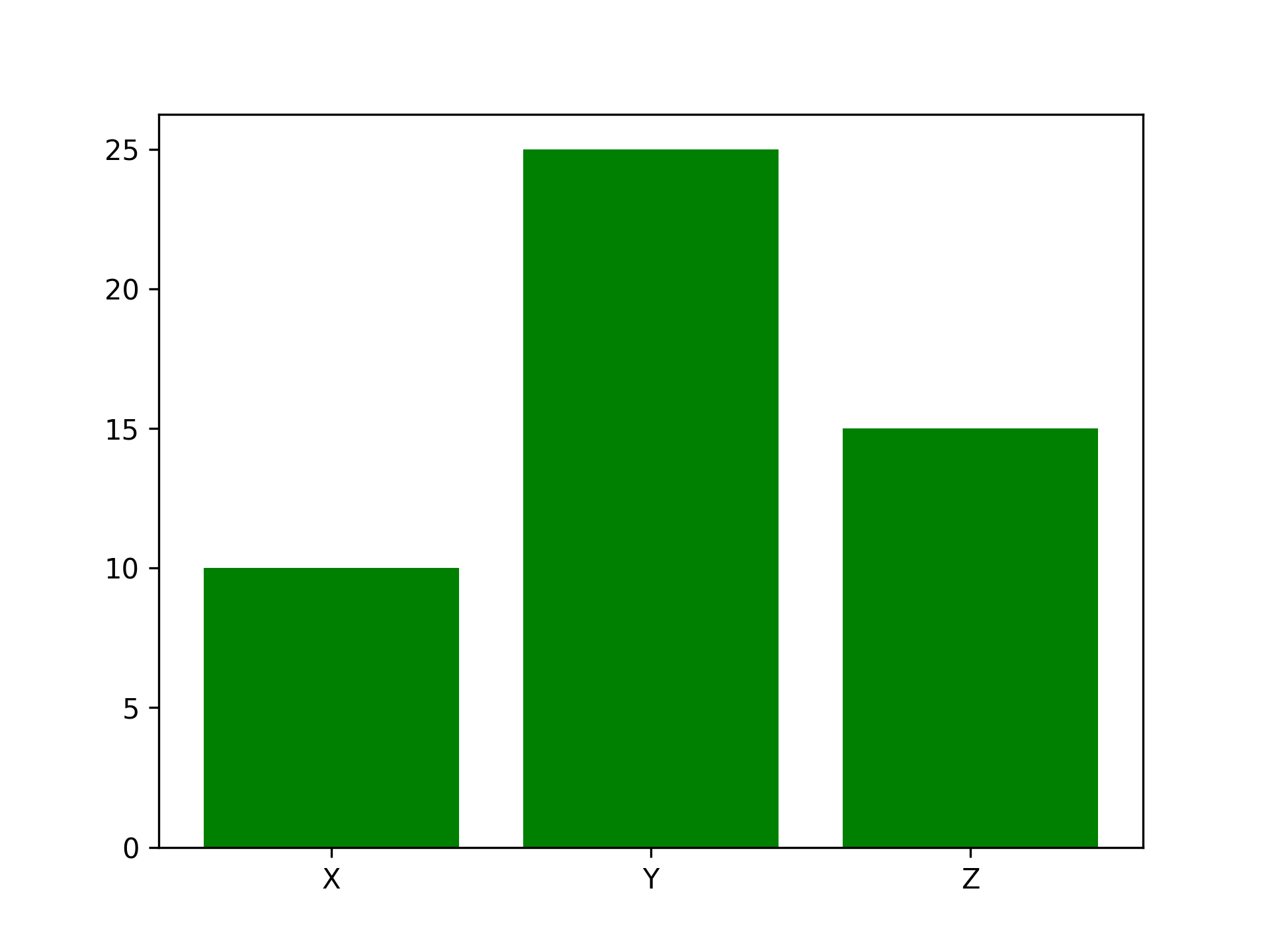# .bar()

The `.bar()` function returns a chart/graph that represents categorical data using vertical bars with heights proportional to the values that they represent. Bars can be plotted as stacked or grouped.

## Syntax

``````plt.bar(x, height, width, bottom, align, kwargs)
``````

The parameters to the function are:

• `x`: Float or array-like values (may be an index and is not required to be scalar).
• `height`: Float or array-like values designating the heights of the bars.
• `width`: Float or array-like values designating the widths of the bars (the default is 0.8).
• `bottom`: Float or array-like values to designate the y coordinates of the bars (default: 0).
• `align`: Keyword (‘center’ or ‘edge’) designating the bar alignment (default: ‘center’).
• `kwargs`: Keywords (properties of Matplotlib rectangle) can be passed to further customize elements of the plot (e.g. fill, color, hatch).

## Example

Goal: Return the value of “X”, “Y” and “Z” in a graph representation. In this example, the `x` and `y` arrays will be allocated to the `x` and `height` arguments respectively. The last argument specifies the color of the bars.

```import matplotlib.pyplot as pltimport numpy as np
x = np.array(["X", "Y", "Z"])y = np.array([10, 25, 15])
plt.bar(x, y, color = "green")plt.show()
```

Output: